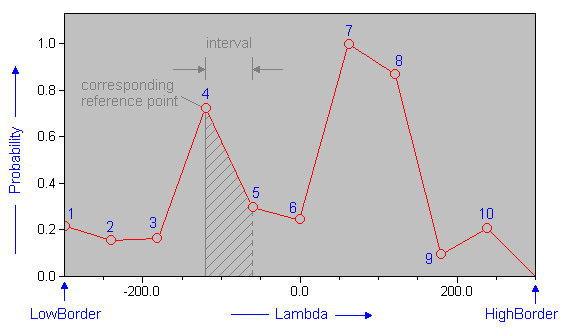The SDL Component Suite is an industry leading collection of components supporting scientific and engineering computing. Please visit the SDL Web site for more information....## How to use TRandGen

The configurable random generator RandGen returns random numbers of a predefined distribution. The user may define the shape of the probability distribution as well as the range of the random numbers. The shape of the probability distribution function is defined by a series of reference points. The number of reference points can be adjusted by the property Resolution and may take any value between 10 and 4000 points. The default value of 500 is sufficiently accurate for most purposes.

Random numbers can be generated by calling the method random. The range of the generated random numbers is defined by the properties LowBorder and HighBorder. Numbers less than LowBorder and greater than HighBorder have a zero probability to be generated. The probabilities of the random numbers within the specified range are defined by the array property Probability. The index of this property runs from 1 to Resolution and defines the evenly spaced reference points of the probability distribution. The reference points can be set up either by loading the Probability array, or by calling one of the built-in distribution functions (i.e. NormalDistri, or UniformDistri). After changing the Probability property, the method Normalize should be called in order to ensure that the probabilities are scaled to values between 0.0 and 1.0. The x-value associated with a given reference point can be obtained by the read-only property Lambda.

The figure below shows, as an example, the distribution function created by only 10 reference points between -300 and +300. Please note, that the range defined by LowBorder and HighBorder is divided into Resolution (10) intervals. The reference points are located at the lower side of each interval. The probability within one interval is interpolated between its reference point and the reference point of the next interval. The probability of the last interval is extrapolated towards zero.Here's a simple example how to apply the random generator (assuming that you have put a TRandGen instance from the VCL palette to the form):

```var
rn      : double;
i       : integer;

// assume that the properties Resolution, LowBorder, and HighBorder
// have been set in the Object Inspector as follows:
// Resolution: 1000, LowBorder: 0, HighBorder: 100

begin
RandGen.NormalDistri (50,15); { define a normal distribution }
for i:=1 to 200 do
begin
rn := RandGen.Random; { create 200 random numbers }
(*... process random numbers ...*) { and process them }
end;
end;
```

Last Update: 2013-May-14